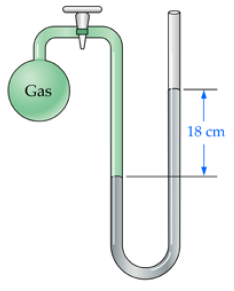# Problem: What is the pressure (in atmospheres) of the gas inside the container connected to an open-end, mercury-filled manometer as shown in the picture? The atmospheric pressure is 0.95 atm.Express your answer numerically in atmospheres.Gases exert a measurable pressure (P) on the walls of their container. The SI unit for pressure is the pascal (Pa). Other pressure units frequently used in chemistry are millimeters of mercury (mmHg) and atmospheres (atm). You can convert between units of pressure using conversion factors such as those indicated below. 1 atm = 760 mmHg = 101, 325 Pa

###### FREE Expert SolutionView Complete Written Solution
###### Problem Details

What is the pressure (in atmospheres) of the gas inside the container connected to an open-end, mercury-filled manometer as shown in the picture? The atmospheric pressure is 0.95 atm.Gases exert a measurable pressure (P) on the walls of their container. The SI unit for pressure is the pascal (Pa). Other pressure units frequently used in chemistry are millimeters of mercury (mmHg) and atmospheres (atm). You can convert between units of pressure using conversion factors such as those indicated below. 1 atm = 760 mmHg = 101, 325 Pa